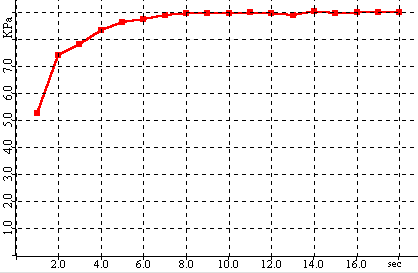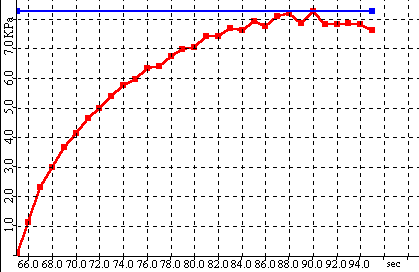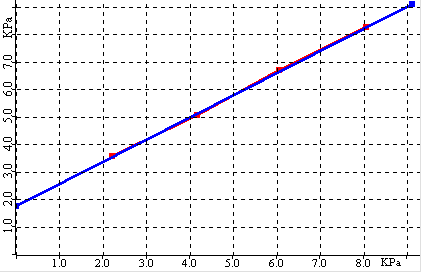# Yield Locus Analysis

 Sample Consolidation Normal Load versus TimeIn the steady state step, shear stress is applied to the sample until the measured shear force and sample volume become stable. With linear cells, the shear stress is applied by moving pushing the lower ring of the cell at a fixed rate relative to the upper ring.For rotational cells, the lid is rotated a fixed rate. The steady state point is the point at which the shear force becomes stable.At the steady state point, the sample has reached a repeatable, stable density relative to the applied compressive load.

 Steady State Shear Force versus TimeIn the analysis step, the shear stress is reduced to zero by reversing the shear stress mechanism.The normal load is then reduced to a predetermined level called the shear load and the shear stress is again applied.The shear forces rises as the sample resists shearing until a maximum shear force is reached.At this point the sample fails and the shear force drops rapidly.The generate yield point consists of the maximum shear force and the shear load.

 Static Failure Analysis Shear Force versus TimeBy repeating the above sequence 3 to 5 times, a series of yield points are generated from which a yield locus can be plotted.The yield points are selected so that they are in the linear portion of the yield locus.

 Static Failure Points Shear Force versus TimeA least squares regression is performed to calculate a linear function for the yield locus.The slope of the calculated line is the angle of internal friction.The intercept of the line is the cohesion.From the cohesion, angle of internal friction and steady state point, the unconfined yield strength and major consolidation stress are calculated using Mohr Coulomb equations.

 Static Yield Locus Shear Force versus Normal LoadBecause the yield points are generated by measuring several steady states for the same sample, the steady state point used for the strength calculation is the average of all the steady state points.In addition, to account for the effect of the steady state on the measured shear force during failure analysis, the measured shear force can be adjusted based on whether its steady state was higher or lower than the average.This is called prorating and can correct for variations in sample density for each yield point measurement.

Compressibility is calculated using the sample’s initial density and density after the consolidation step.

Static yield analysis generates the strength of a static or not-moving sample.This would be the condition in a silo or chute when the sample is at rest.Therefore, to get the sample to flow, the force used to move the sample must be greater than the static yield strength.